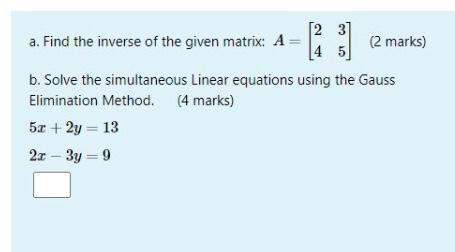### Create an Account

Home / Questions /  a. Find the inverse of the given matrix: A (2 marks) 4 5 b. Solve the simultaneous Li...

#  a. Find the inverse of the given matrix: A (2 marks) 4 5 b. Solve the simultaneous Linear equations using the Gauss Elimination Method. (4 marks) 5x + 2y = 13 2x - 3y = 9

 a. Find the inverse of the given matrix: A (2 marks) 4 5 b. Solve the simultaneous Linear equations using the Gauss Elimination Method. (4 marks) 5x + 2y = 13 2x - 3y = 9May 06 2021 View more View LessSubscribe To Get Solution